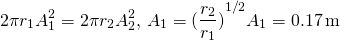16 Waves

16.4 Energy and Power of a Wave

Learning Objectives

By the end of this section, you will be able to:

• Explain how energy travels with a pulse or wave
• Describe, using a mathematical expression, how the energy in a wave depends on the amplitude of the wave

All waves carry energy, and sometimes this can be directly observed. Earthquakes can shake whole cities to the ground, performing the work of thousands of wrecking balls ((Figure)). Loud sounds can pulverize nerve cells in the inner ear, causing permanent hearing loss. Ultrasound is used for deep-heat treatment of muscle strains. A laser beam can burn away a malignancy. Water waves chew up beaches.Figure 16.15 The destructive effect of an earthquake is observable evidence of the energy carried in these waves. The Richter scale rating of earthquakes is a logarithmic scale related to both their amplitude and the energy they carry.

In this section, we examine the quantitative expression of energy in waves. This will be of fundamental importance in later discussions of waves, from sound to light to quantum mechanics.

Energy in Waves

The amount of energy in a wave is related to its amplitude and its frequency. Large-amplitude earthquakes produce large ground displacements. Loud sounds have high-pressure amplitudes and come from larger-amplitude source vibrations than soft sounds. Large ocean breakers churn up the shore more than small ones. Consider the example of the seagull and the water wave earlier in the chapter ((Figure)). Work is done on the seagull by the wave as the seagull is moved up, changing its potential energy. The larger the amplitude, the higher the seagull is lifted by the wave and the larger the change in potential energy.

The energy of the wave depends on both the amplitude and the frequency. If the energy of each wavelength is considered to be a discrete packet of energy, a high-frequency wave will deliver more of these packets per unit time than a low-frequency wave. We will see that the average rate of energy transfer in mechanical waves is proportional to both the square of the amplitude and the square of the frequency. If two mechanical waves have equal amplitudes, but one wave has a frequency equal to twice the frequency of the other, the higher-frequency wave will have a rate of energy transfer a factor of four times as great as the rate of energy transfer of the lower-frequency wave. It should be noted that although the rate of energy transport is proportional to both the square of the amplitude and square of the frequency in mechanical waves, the rate of energy transfer in electromagnetic waves is proportional to the square of the amplitude, but independent of the frequency.

Power in Waves

Consider a sinusoidal wave on a string that is produced by a string vibrator, as shown in (Figure). The string vibrator is a device that vibrates a rod up and down. A string of uniform linear mass density is attached to the rod, and the rod oscillates the string, producing a sinusoidal wave. The rod does work on the string, producing energy that propagates along the string. Consider a mass element of the string with a mass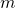, as seen in (Figure). As the energy propagates along the string, each mass element of the string is driven up and down at the same frequency as the wave. Each mass element of the string can be modeled as a simple harmonic oscillator. Since the string has a constant linear density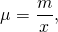each mass element of the string has the mass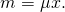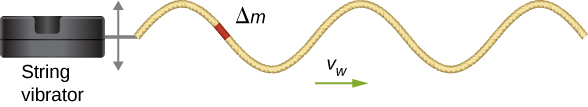Figure 16.16 A string vibrator is a device that vibrates a rod. A string is attached to the rod, and the rod does work on the string, driving the string up and down. This produces a sinusoidal wave in the string, which moves with a wave velocity v. The wave speed depends on the tension in the string and the linear mass density of the string. A section of the string with mass     oscillates at the same frequency as the wave.

The total mechanical energy of the wave is the sum of its kinetic energy and potential energy. The kinetic energy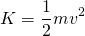of each mass element of the string of length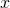is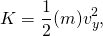as the mass element oscillates perpendicular to the direction of the motion of the wave. Using the constant linear mass density, the kinetic energy of each mass element of the string with lengthis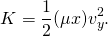A differential equation can be formed by letting the length of the mass element of the string approach zero,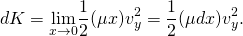Since the wave is a sinusoidal wave with an angular frequency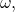the position of each mass element may be modeled as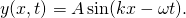Each mass element of the string oscillates with a velocity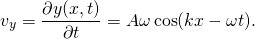The kinetic energy of each mass element of the string becomes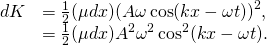The wave can be very long, consisting of many wavelengths. To standardize the energy, consider the kinetic energy associated with a wavelength of the wave. This kinetic energy can be integrated over the wavelength to find the energy associated with each wavelength of the wave: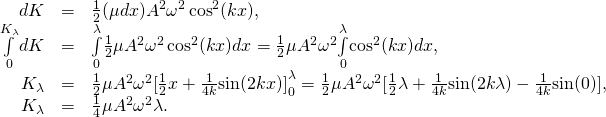There is also potential energy associated with the wave. Much like the mass oscillating on a spring, there is a conservative restoring force that, when the mass element is displaced from the equilibrium position, drives the mass element back to the equilibrium position. The potential energy of the mass element can be found by considering the linear restoring force of the string, In Oscillations, we saw that the potential energy stored in a spring with a linear restoring force is equal towhere the equilibrium position is defined as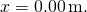When a mass attached to the spring oscillates in simple harmonic motion, the angular frequency is equal to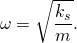As each mass element oscillates in simple harmonic motion, the spring constant is equal to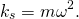The potential energy of the mass element is equal to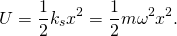Note that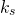is the spring constant and not the wave number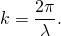This equation can be used to find the energy over a wavelength. Integrating over the wavelength, we can compute the potential energy over a wavelength: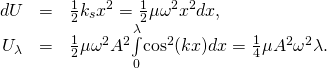The potential energy associated with a wavelength of the wave is equal to the kinetic energy associated with a wavelength.

The total energy associated with a wavelength is the sum of the potential energy and the kinetic energy: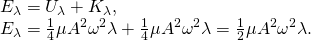The time-averaged power of a sinusoidal mechanical wave, which is the average rate of energy transfer associated with a wave as it passes a point, can be found by taking the total energy associated with the wave divided by the time it takes to transfer the energy. If the velocity of the sinusoidal wave is constant, the time for one wavelength to pass by a point is equal to the period of the wave, which is also constant. For a sinusoidal mechanical wave, the time-averaged power is therefore the energy associated with a wavelength divided by the period of the wave. The wavelength of the wave divided by the period is equal to the velocity of the wave,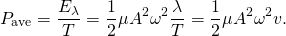Note that this equation for the time-averaged power of a sinusoidal mechanical wave shows that the power is proportional to the square of the amplitude of the wave and to the square of the angular frequency of the wave. Recall that the angular frequency is equal to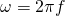, so the power of a mechanical wave is equal to the square of the amplitude and the square of the frequency of the wave.

Example

Power Supplied by a String Vibrator

Consider a two-meter-long string with a mass of 70.00 g attached to a string vibrator as illustrated in (Figure). The tension in the string is 90.0 N. When the string vibrator is turned on, it oscillates with a frequency of 60 Hz and produces a sinusoidal wave on the string with an amplitude of 4.00 cm and a constant wave speed. What is the time-averaged power supplied to the wave by the string vibrator?

Strategy

The power supplied to the wave should equal the time-averaged power of the wave on the string. We know the mass of the string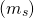, the length of the string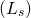, and the tension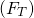in the string. The speed of the wave on the string can be derived from the linear mass density and the tension. The string oscillates with the same frequency as the string vibrator, from which we can find the angular frequency.

Solution

1. Begin with the equation of the time-averaged power of a sinusoidal wave on a string:The amplitude is given, so we need to calculate the linear mass density of the string, the angular frequency of the wave on the string, and the speed of the wave on the string.

2. We need to calculate the linear density to find the wave speed: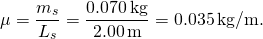3. The wave speed can be found using the linear mass density and the tension of the string: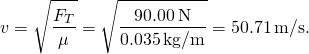4. The angular frequency can be found from the frequency: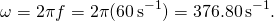5. Calculate the time-averaged power: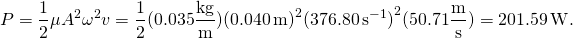Significance

The time-averaged power of a sinusoidal wave is proportional to the square of the amplitude of the wave and the square of the angular frequency of the wave. This is true for most mechanical waves. If either the angular frequency or the amplitude of the wave were doubled, the power would increase by a factor of four. The time-averaged power of the wave on a string is also proportional to the speed of the sinusoidal wave on the string. If the speed were doubled, by increasing the tension by a factor of four, the power would also be doubled.

Is the time-averaged power of a sinusoidal wave on a string proportional to the linear density of the string?

[hidden-answer a=”671846″]At first glance, the time-averaged power of a sinusoidal wave on a string may look proportional to the linear density of the string because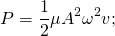however, the speed of the wave depends on the linear density. Replacing the wave speed with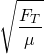shows that the power is proportional to the square root of tension and proportional to the square root of the linear mass density: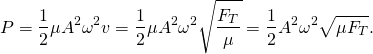The equations for the energy of the wave and the time-averaged power were derived for a sinusoidal wave on a string. In general, the energy of a mechanical wave and the power are proportional to the amplitude squared and to the angular frequency squared (and therefore the frequency squared).

Another important characteristic of waves is the intensity of the waves. Waves can also be concentrated or spread out. Waves from an earthquake, for example, spread out over a larger area as they move away from a source, so they do less damage the farther they get from the source. Changing the area the waves cover has important effects. All these pertinent factors are included in the definition of intensity (I) as power per unit area: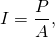where P is the power carried by the wave through area A. The definition of intensity is valid for any energy in transit, including that carried by waves. The SI unit for intensity is watts per square meter (W/m2). Many waves are spherical waves that move out from a source as a sphere. For example, a sound speaker mounted on a post above the ground may produce sound waves that move away from the source as a spherical wave. Sound waves are discussed in more detail in the next chapter, but in general, the farther you are from the speaker, the less intense the sound you hear. As a spherical wave moves out from a source, the surface area of the wave increases as the radius increases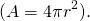The intensity for a spherical wave is therefore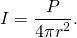If there are no dissipative forces, the energy will remain constant as the spherical wave moves away from the source, but the intensity will decrease as the surface area increases.

In the case of the two-dimensional circular wave, the wave moves out, increasing the circumference of the wave as the radius of the circle increases. If you toss a pebble in a pond, the surface ripple moves out as a circular wave. As the ripple moves away from the source, the amplitude decreases. The energy of the wave spreads around a larger circumference and the amplitude decreases proportional to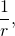and not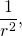as in the case of a spherical wave.

Summary

• The energy and power of a wave are proportional to the square of the amplitude of the wave and the square of the angular frequency of the wave.
• The time-averaged power of a sinusoidal wave on a string is found by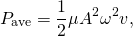where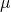is the linear mass density of the string, A is the amplitude of the wave,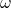is the angular frequency of the wave, and v is the speed of the wave.

• Intensity is defined as the power divided by the area. In a spherical wave, the area is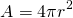and the intensity isAs the wave moves out from a source, the energy is conserved, but the intensity decreases as the area increases.

Conceptual Questions

Consider a string with under tension with a constant linear mass density. A sinusoidal wave with an angular frequency and amplitude produced by some external driving force. If the frequency of the driving force is decreased to half of the original frequency, how is the time-averaged power of the wave affected? If the amplitude of the driving force is decreased by half, how is the time-averaged power affected? Explain your answer.

The time averaged power is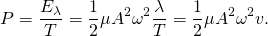If the frequency or amplitude is halved, the power decreases by a factor of 4.

Circular water waves decrease in amplitude as they move away from where a rock is dropped. Explain why.

In a transverse wave on a string, the motion of the string is perpendicular to the motion of the wave. If this is so, how is possible to move energy along the length of the string?

[hidden-answer a=”806307″]As a portion on the string moves vertically, it exerts a force on the neighboring portion of the string, doing work on the portion and transferring the energy.[/hidden-answer]

The energy from the sun warms the portion of the earth facing the sun during the daylight hours. Why are the North and South Poles cold while the equator is quite warm?

The intensity of a spherical waves decreases as the wave moves away from the source. If the intensity of the wave at the source is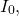how far from the source will the intensity decrease by a factor of nine?
[hidden-answer a=”98685″]The intensity of a spherical wave is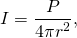if no energy is dissipated the intensity will decrease by a factor of nine at three meters.[/hidden-answer]

Problems

A string of length 5 m and a mass of 90 g is held under a tension of 100 N. A wave travels down the string that is modeled as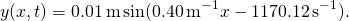What is the power over one wavelength?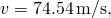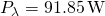Ultrasound of intensity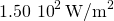is produced by the rectangular head of a medical imaging device measuring 3.00 cm by 5.00 cm. What is its power output?

The low-frequency speaker of a stereo set has a surface area of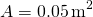and produces 1 W of acoustical power. (a) What is the intensity at the speaker? (b) If the speaker projects sound uniformly in all directions, at what distance from the speaker is the intensity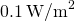?

a.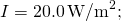b.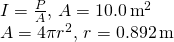To increase the intensity of a wave by a factor of 50, by what factor should the amplitude be increased?

A device called an insolation meter is used to measure the intensity of sunlight. It has an area of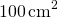and registers 6.50 W. What is the intensity in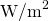?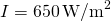Energy from the Sun arrives at the top of Earth’s atmosphere with an intensity of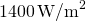. How long does it take for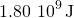to arrive on an area of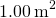?

Suppose you have a device that extracts energy from ocean breakers in direct proportion to their intensity. If the device produces 10.0 kW of power on a day when the breakers are 1.20 m high, how much will it produce when they are 0.600 m high?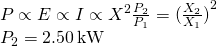A photovoltaic array of (solar cells) isefficient in gathering solar energy and converting it to electricity. If the average intensity of sunlight on one day is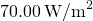, what area should your array have to gather energy at the rate of 100 W? (b) What is the maximum cost of the array if it must pay for itself in two years of operation averaging 10.0 hours per day? Assume that it earns money at the rate of 9.00 cents per kilowatt-hour.

A microphone receiving a pure sound tone feeds an oscilloscope, producing a wave on its screen. If the sound intensity is originally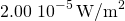, but is turned up until the amplitude increases by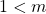, what is the new intensity?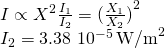A string with a mass of 0.30 kg has a length of 4.00 m. If the tension in the string is 50.00 N, and a sinusoidal wave with an amplitude of 2.00 cm is induced on the string, what must the frequency be for an average power of 100.00 W?

The power versus time for a point on a string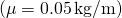in which a sinusoidal traveling wave is induced is shown in the preceding figure. The wave is modeled with the wave equation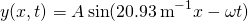. What is the frequency and amplitude of the wave?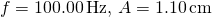A string is under tension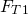. Energy is transmitted by a wave on the string at rate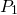by a wave of frequency. What is the ratio of the new energy transmission rate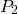toif the tension is doubled?

A 250-Hz tuning fork is struck and the intensity at the source is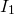at a distance of one meter from the source. (a) What is the intensity at a distance of 4.00 m from the source? (b) How far from the tuning fork is the intensity a tenth of the intensity at the source?

a.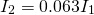; b.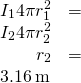A sound speaker is rated at a voltage of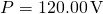and a current of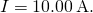Electrical power consumption is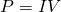. To test the speaker, a signal of a sine wave is applied to the speaker. Assuming that the sound wave moves as a spherical wave and that all of the energy applied to the speaker is converted to sound energy, how far from the speaker is the intensity equal to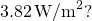The energy of a ripple on a pond is proportional to the amplitude squared. If the amplitude of the ripple is 0.1 cm at a distance from the source of 6.00 meters, what was the amplitude at a distance of 2.00 meters from the source?